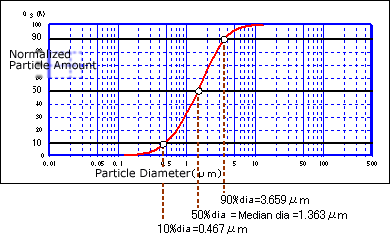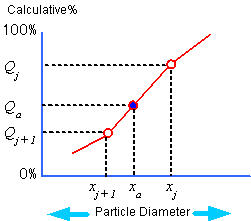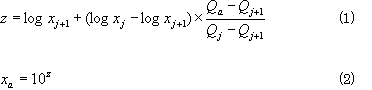# Arbitrary % Particle Size and Median Diameter

Though particle size distribution data is expressed as cumulative % or frequency % against particle size scale, it is also sometimes inversely expressed as particle size against cumulative % scale.

As shown in Fig. 1, the particle sizes at the points where the cumulative % distribution curve intersects the horizontal axis at the 10 %, 50 % and 90 % positions are called "10 % diameter," "50 % diameter," and "90 % diameter," respectively. 10 %, 50 % and 90 % are not especially fixed positions; any cumulative % is used as necessary. This is called the "arbitrary % particle size."Fig. 1

The 50 % particle size is also called the "median diameter" and is very generally used.

When comparing the size of the particle size distribution of two or more samples, this median diameter is often used since the size of the measurement target must be represented by a single numerical value. Therefore, median diameter is often confused with the average particle size. However, these two sizes having different definitions normally do not match. These two match only when the particle size distribution is symmetrical about the center (50 % diameter).

Fig. 2 and formulas (1) and (2) below show how to calculate the arbitrary % particle size.Fig. 2First, let's calculate arbitrary particle size as xa at cumulative % Qa.

When cumulative % Qj+1 and Qj at the two particle sizes xj+1 and xj, which are at both sides of this xa, are already known, arbitrary % particle size xa at cumulative % Qa can be calculated using formulas (1) and (2).

If calculation is done with Qa as 50 %, then the median diameter (= 50 % diameter) can be obtained.